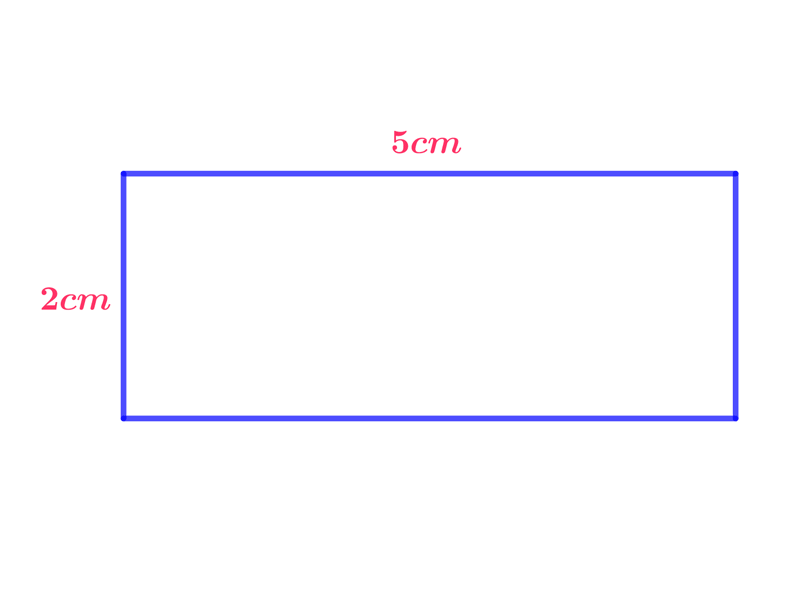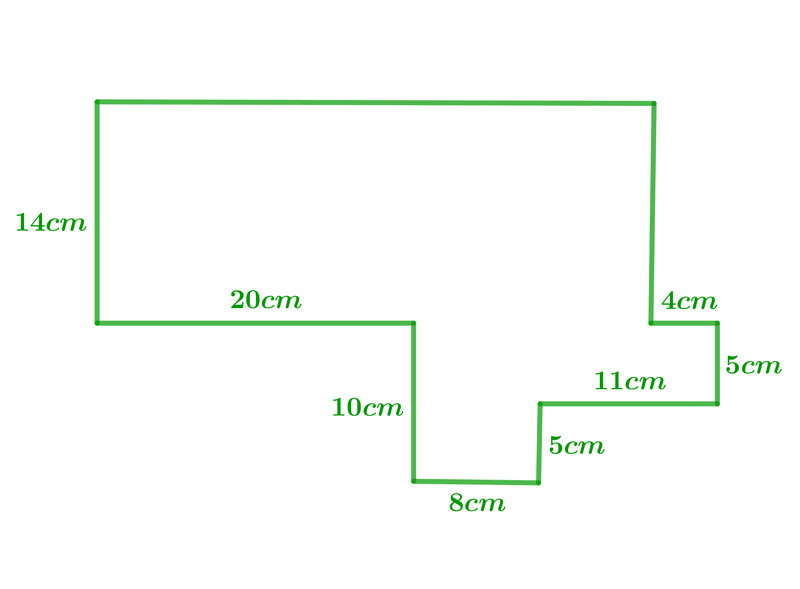Math Calculators, Lessons and Formulas

It is time to solve your math problem

mathportal.org
• Pre algebra
• Whole numbers
• Operations with whole numbers

# Operations with whole numbers

ans:
syntax error
C
DEL
ANS
±
(
)
÷
×
7
8
9
4
5
6
+
1
2
3
=
0
.
auto next question
calculator
•  Question 1: 1 pts The statement $4+(1+2)=(4+1)+2$ illustrates which property of addition?
 Identity property Associative property Commutative property none of these
•  Question 2: 1 pts Caleb wants 100 new pens. Each one costs $2.$ How much will he spend?
 $20$ $200$ $2000$ $20000$
•  Question 3: 1 pts The statement $5\cdot 7= 7\cdot 5$ is an illustration of which property of multiplication?
 Commutative property Identity property Associative property Zero factor property
•  Question 4: 2 pts Calculate:
$5+3\cdot 7=$
•  Question 5: 2 pts Calculate $2^{5}\div 2+2\cdot3+ (5-3-1)$
 20 21 22 23
•  Question 6: 2 pts Find the perimeter and area of the following figure.$O=9 cm, P=10cm^{2}$ $O=14 cm, P=20cm^{2}$ $O=14 cm, P=10cm^{2}$ $O=12 cm, P=10cm^{2}$
•  Question 7: 2 pts Some number multiplied by 19 gives a product of 551. Which expression can be used to find unknown number
 $x\cdot 19=551$ $x\div 19=551$ $x+19=551$ $19\div x=551$
•  Question 8: 2 pts Suppose that you need a total of $380$ points on four tests during the semester to receive an A for the course. Your scores on the first three tests were $100, 99,$ and $101.$ What is the lowest score you can get on the fourth test and still receive the A?
 $100$ $90$ $80$ $70$
•  Question 9: 3 pts Find the perimeter.$O=127 cm$ $O=126 cm$ $O=125 cm$ $O=124 cm$
•  Question 10: 3 pts The fifth grade students are going on a field trip. Of the 87 students, 72 of them will ride the buses. The remainder of them will ride in cars that can hold up to four students each. Which expression can be used to find the number of cars needed?
 $\frac{87+72}{4}$ $87-\frac{72}{4}$ $\frac{87-72}{4}$ $87+\frac{72}{4}$
•  Question 11: 3 pts The length of a road is 213 feet. John wants to plant trees 18 feet apart along the road. John estimates that he can plant 13 trees. Is he correct and how do you know?
 Yes he is correct because 18 trees at 13 feet apart would fit along a 213 ft. road. Yes he is correct because 13 trees at 18 feet apart would fit along a 213 ft. road. No he is not correct because 13 trees at 18 feet apart would take more than 213 ft. No he is not correct because more than 13 trees would fit on a 213 foot road.
•  Question 12: 3 pts Which expression shows "the difference of 9 and 3 divided by 2 multiplied by 8"?
 $9-3\div (2 \cdot 8)$ $(9-3)\div 2 \cdot 8$ $2\cdot 8- 9\div 3$ $9-3\div 2 \cdot 8$